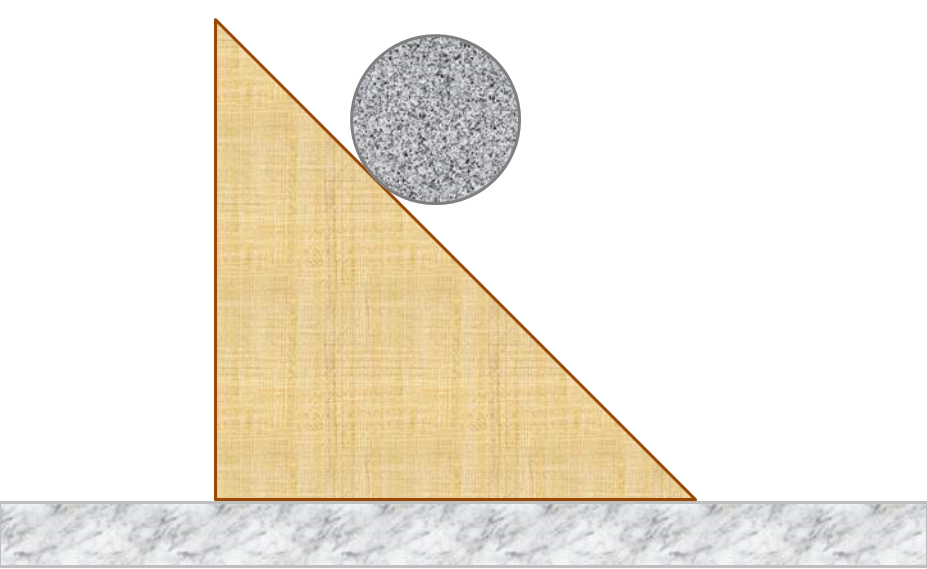# Rolling BallA uniform solid ball of mass $m$ rolls without slipping down a wedge of mass $2m$ and angle $45^\circ$, which itself can slide without friction on a horizontal floor. The acceleration of the ball relative to the wedge can be expressed as $\dfrac{p}{q}\sqrt{2}g$, where $g$ denotes the gravitational acceleration. Calculate $q-p$.

×